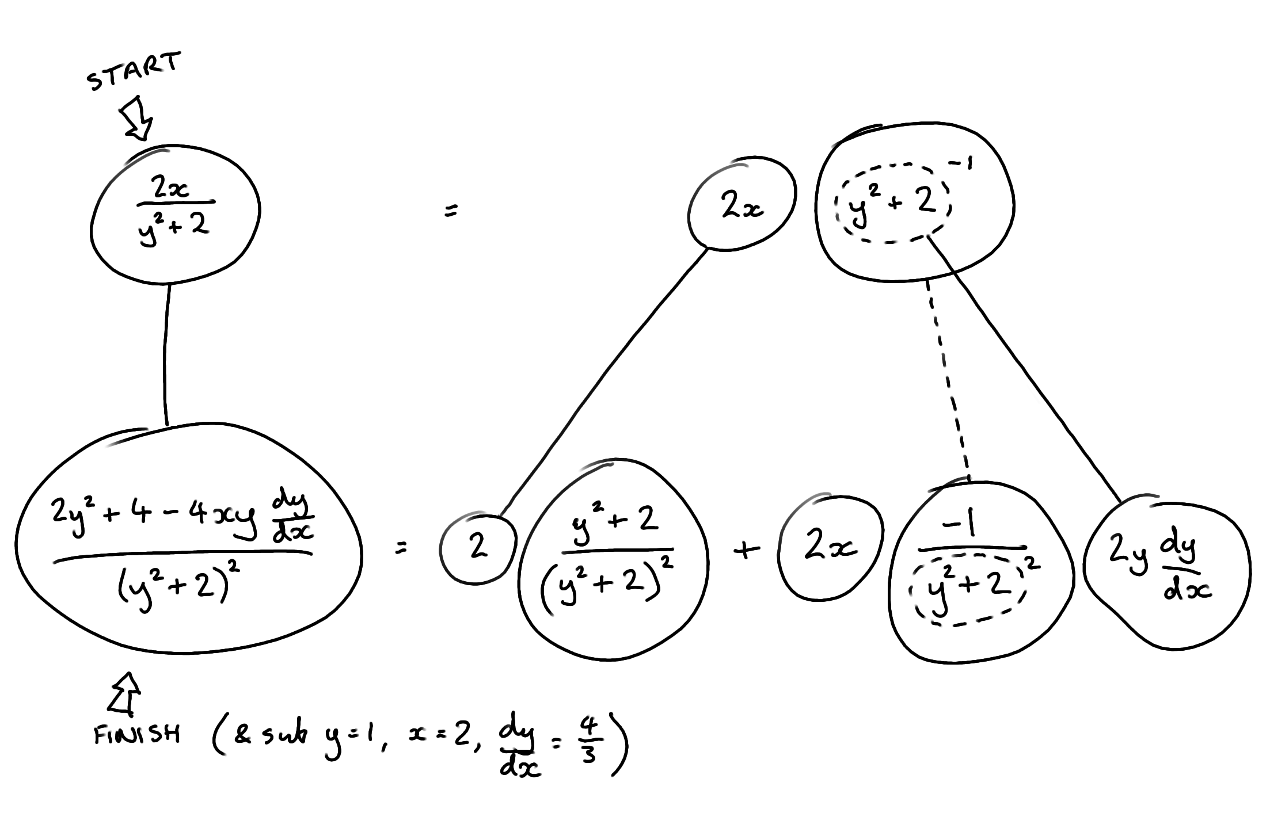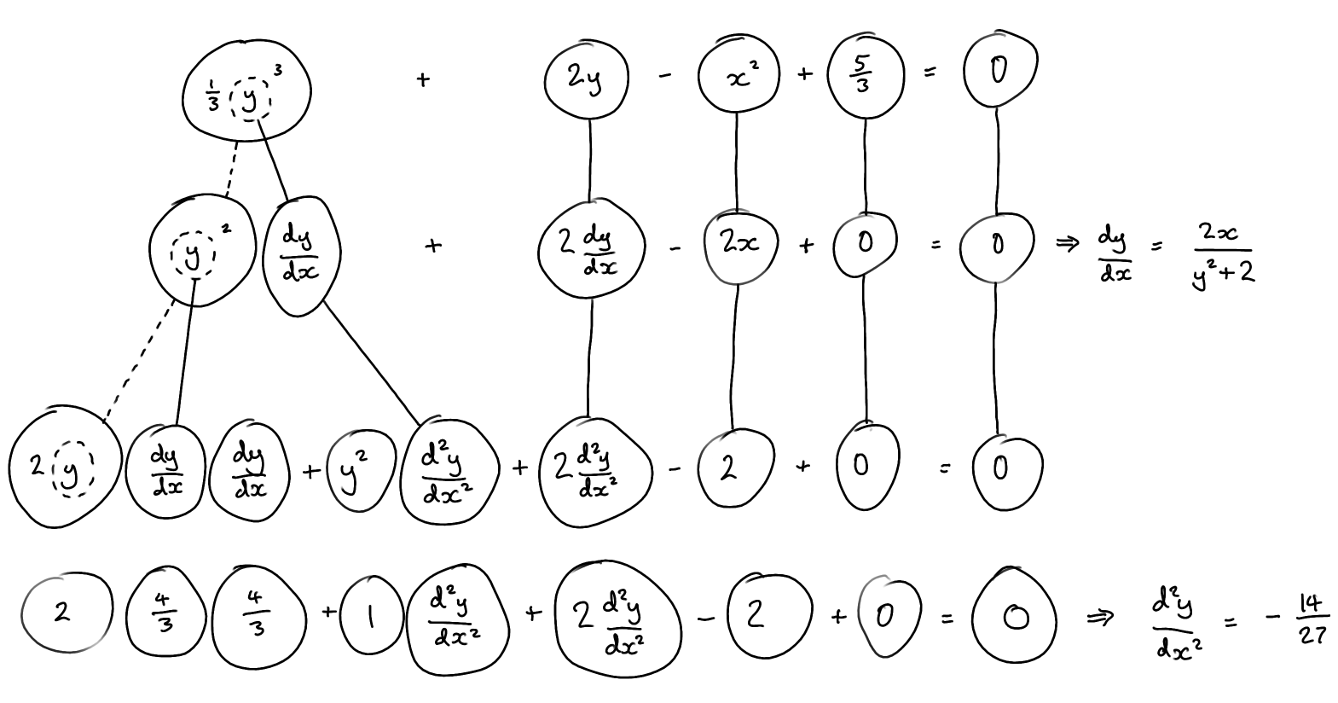# Thread: Second Derivative Value via Implicit Differentiation

1. ## Second Derivative Value via Implicit Differentiation

Hi guys, I haven't posted in a while but I was tutoring someone and ran into a problem that I thought had got right but blackboard said was incorrect.

The problem is

$\displaystyle \frac{y^{3}}{3} +2y -x^2 + \frac{5}{3} = 0$

Now find the second derivative when x = 2 and y =1

I keep getting 3/4 as my answer. I solved it using implicit differentiation and also using partials differentiation to double check. The correct answer according to blackboard is -14/27

and can someone show me what im doing wrong with my latex. Guess im a bit rusty. Thanks

2. ## re: Second Derivative Value via Implicit DifferentiationOriginally Posted by 11rdc11and can someone show me what im doing wrong with my latex. Guess im a bit rusty. Thanks
Use "tex" tags, "math" tags are no good here at the moment

$\displaystyle \displaystyle \frac{y^3}{3}+2y-x^2 + \frac{5}{3} = 0$

3. ## re: Second Derivative Value via Implicit DifferentiationOriginally Posted by 11rdc11Hi guys, I haven't posted in a while but I was tutoring someone and ran into a problem that I thought had got right but blackboard said was incorrect.

The problem is

$\displaystyle \frac{y^{3}}{3} +2y -x^2 + \frac{5}{3} = 0$

Now find the second derivative when x = 2 and y =1

I keep getting 3/4 as my answer. I solved it using implicit differentiation and also using partials differentiation to double check. The correct answer according to blackboard is -14/27

and can someone show me what im doing wrong with my latex. Guess im a bit rusty. Thanks
Your function is defined by $\displaystyle \frac{y^{3}}{3} +2y -x^2 + \frac{5}{3} = 0$ . Deriving: $\displaystyle y^2y'+2y'-2x=0$ . Substituting $\displaystyle x=2,y=1$ we get $\displaystyle 3y'-4=0$ or equivalently $\displaystyle y'=4/3$ . Derive again and substitute $\displaystyle x=2,y=1,y'=4/3$ .

4. ## re: Second Derivative Value via Implicit Differentiation

Thanks guys but even when i take the second derivative i get the same

$\displaystyle y' = \frac{2x}{y^2+2}$

now taking the derivative again

$\displaystyle y'' = \frac{\frac{2}{y^2+2}}{\frac{4xy}{(y^2+2)^2}}$

which simplifies to

$\displaystyle y'' = \frac{y^2+2}{2xy}$

and plugging in the values you 3/4 right?

5. ## re: Second Derivative Value via Implicit DifferentiationOriginally Posted by 11rdc11$\displaystyle y'' = \frac{y^2+2}{2xy}$

and plugging in the values you 3/4 right?
Looks good to me.

6. ## re: Second Derivative Value via Implicit Differentiation

Thanks that is what I thought but it kept telling me that I was wrong.

7. ## re: Second Derivative Value via Implicit Differentiation

Deriving $\displaystyle y^2y'+2y'-2x=0$ we get $\displaystyle 2y(y')^2+y^2y''+2y''-2=0$ . Substituting $\displaystyle x=2,y=1,y'=4/3$ you'll obtain $\displaystyle y''(2)=-14/27$ .

8. ## re: Second Derivative Value via Implicit DifferentiationOriginally Posted by pickslidesUse "tex" tags, "math" tags are no good here at the moment

$\displaystyle \displaystyle \frac{y^3}{3}+2y-x^2 + \frac{5}{3} = 0$
You mean "Jedi math tags do not work on MHF ony tex..."

CB

9. ## Re: Second Derivative Value via Implicit DifferentiationOriginally Posted by 11rdc11$\displaystyle y' = \frac{2x}{y^2+2}$

now taking the derivative again

$\displaystyle y'' = \frac{\frac{2}{y^2+2}}{\frac{4xy}{(y^2+2)^2}}$
A point against the quotient rule? Many people prefer to see the quotient as a product, anyway. Just in case a picture helps...... where (key in spoiler) ...

Spoiler:... is the chain rule. Straight continuous lines differentiate downwards (integrate up) with respect to the main variable (in this case x), and the straight dashed line similarly but with respect to the dashed balloon expression (the inner function of the composite which is subject to the chain rule).

But this is wrapped inside the legs-uncrossed version of...... the product rule, where, again, straight continuous lines are differentiating downwards with respect to x.

FR's method is another way of avoiding the quotient rule here. In balloon sculpture...__________________________________________________ __________

Don't integrate - balloontegrate!

Balloon Calculus; standard integrals, derivatives and methods

Balloon Calculus Drawing with LaTeX and Asymptote!

#### Search Tags

derivative, differentiation, implicit